# Molarity Formula

Molarity Formula

Molarity is the most commonly used term to describe the concentration of a solution. It is equal to the moles of solute divided by the liters of solution. The solute is defined as the substance being dissolved, while the solvent is the substance where the solute is dissolved (usually water). The solution is the combination of the solute and the solvent.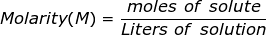Molarity Formula Questions:

1. What is the molarity of a solution if 1.47 moles of sugar is dissolved into 2.31 L of solution?

Answer: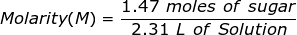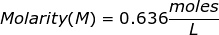2. How many grams of NaCl will be needed to make 1.50 Liters of an aqueous 0.500 M solution?

Answer:

The equation for Molarity must be rearranged to solve for the moles of solute (NaCl). The moles of solute can then be converted into grams.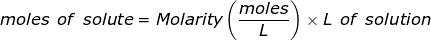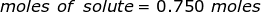Now, convert the moles of solute into grams by using the molar mass of NaCl.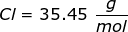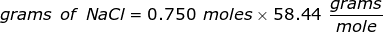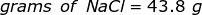Related Links: